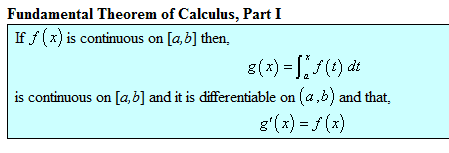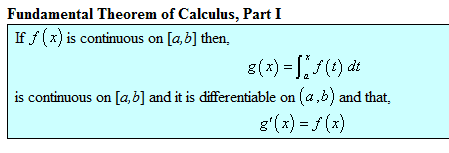# Fundamental Theorem of Calc, Part 1

I'm confused.What's the difference between f(x) and f(t)?

I'm confused.What's the difference between f(x) and f(t)?

I see what you mean, that can be a little confusing.

First, when we talk about f(x), the role of 'x' is that of a dummy variable. If we said f(x) = x^2 or f(t) = t^2, we would be talking about the same function. That's why when people are being picky and pedantic, they say that f is the function; and f(x) is the value of the function at the point x. So the dummy variable has no effect on the function. It's just some variable that ranges over the elements of the domain of f.

Ok so we have some function, which we colloquially call f(x); but it's more proper to think of the function as f. And we're free to use whatever dummy variable we like when we define f or use it as an integrand.

Now we want to define another function, g, by saying that for some point x, g(x) is the definite integral of f from a to x, where a is some fixed constant.

So to evaluate g(x), we compute the integral of f as f ranges between a and x But now we need a dummy variable inside the integral to integrate against. We can't call it x, because we're already using that variable to define g. So we use a new dummy variable, t. We choose an x; then we compute the definite integral of f as f ranges between a and x. We use a new dummy variable 't' to denote that.

Then in the last line where they say g'(x) = f(x), it makes sense to use the same dummy variable, because they're saying that the value of g' and the value of f are the same for any given x.

Perhaps it helps to think of choosing an x_0; then you let t run from a to x_0 and you get a number that's g(x_0). Then you pick another x_1, and let t range over a to x_1, and so forth. That's why you need a separate dummy variable.

Last edited:
HallsofIvy
Homework Helper
Note that you have to have "f(t)" inside the integral because the variable of integration is "t"- which is sometimes referred to as a "dummy" variable (when I was teaching deaf students, I would not use that term!). However,
$$\int_a^x f(t)dt$$
is a function of x, not t. If F(u) is an anti-derivative of f(u) (I have used the variable "u" just to indicate that it really does not matter what symbol you use for the variable) then
$$\int_a^x f(t)dt= F(x)- F(a)$$
a function of x.

So f(x) is what we call the function in normal usage, but when we need to use the variable "x" for something else as is the case in this example, we can use f(t) in f(x)'s stead until we can use the variable "x" again?

HallsofIvy
Homework Helper
Pretty much, yes.

That's... despicable. For understanding's sake it's probably best to just focus on what the theorem is trying to say without getting caught up in the details of how it goes about saying it. Given a continuous, differentiable function, the rate of change of that function's area function is the function itself. Done.

And that makes sense because as the function gets higher and higher, so too does the area bound between it and the x-axis. A small change in the function when f = 9089078907 will produce a huge change in the total area underneath it.

Sorry, just venting my thoughts. Thanks for the replies.

Last edited:
HallsofIvy
Homework Helper
That's... despicable.
what's despicable?

For understanding's sake it's probably best to just focus on what the theorem is trying to say without getting caught up in the details of how it goes about saying it. Given a continuous, differentiable function, the rate of change of that function's area function is the function itself. Done.

And that makes sense because as the function gets higher and higher, so too does the area bound between it and the x-axis. A small change in the function when f = 9089078907 will produce a huge change in the total area underneath it.

Sorry, just venting my thoughts. Thanks for the replies.

Fredrik
Staff Emeritus
Gold Member
That's why when people are being picky and pedantic, they say that f is the function; and f(x) is the value of the function at the point x.
Hey, i was just going to say exactly that.(But I don't consider it pedantic. I think the other terminology is really sloppy and causes a lot of unnecessary confusion).

JamesGold, I even refuse to use phrases like "f(x) is continous". f(x) is a number in the range of the function f, and a number can't be continuous. This is how I would state the theorem in the image you posted:

If f is continous on [a,b], then the function g defined by $$g(x)=\int_a^x f(t)dt$$ for all x≥a, is continuous on [a,b], differentiable on (a,b), and satisfies g'(x)=f(x) for all x in (a,b).

Note that we could also have said that g is defined by $$g(y)=\int_a^y f(x)dx$$ for all y≥a. The statement defines the same function no matter what variable symbol we use.

lavinia